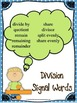# Common Core 5th Grade Math: Writing & Evaluating Numerical Expressions 5.oa.a.2Subject
Resource Type
Common Core State Standards
Product Rating
File Type

PDF (Acrobat) Document File

6 MB|17 pages
Share
Product Description
Print, copy, teach! Everything you need to teach CCSS.5.oa.a.2

This activity packet includes 3 lessons. It also includes math posters and activity cards that will be useful in implementing the lessons. This packet includes the following:

Standard Teaching Notes
Subtraction Signal Words Poster
Multiplication Signal Words Poster
Division Signal Words Poster
Lesson 1: Hands on Models (Teaching Notes)
Lesson 1: Hands on Models (Number Cards Printable)
Lesson 2: Numbers to Words(Teaching Notes)
Lesson 2: Numbers to Words(Worksheet Practice)
Lesson 3: Partner Expression Cards (Teaching Notes)
Lesson 3: Partner Expression Cards (Printable Cards 1)
Lesson 3: Partner Expression Cards (Printable Cards 2)

Prerequisite Skills:
Students should be familiar with addition, subtraction, multiplication, and division. Students should also be aware of the order of operations used in math. (parenthesis, exponents, multiplication, division, addition, subtraction)

Common Core Alignment:
CCSS.MATH.CONTENT.5.OA.A.2Write simple expressions that record calculations with numbers, and interpret numerical expressions without evaluating them.

Activity Objectives:
Given a situation described in words, students will write simple numerical expressions.

Essential Question:
How can I take a word problem and turn it into a numerical expression?

After the teacher models how to turn word problems into numerical expressions, the signal word posters should be explained to the students. The following lessons are then recommended.

Lesson One: “Hands On Models”
The teacher writes the sample word problems on the board. Students use the number cards to construct concrete models of simple numerical expressions. Can be used as an independent task or as partner/group work.

Lesson Two: “Numbers to Words”
The teacher will provide an example of a numerical expression on the board (included in this resource). The teacher will model and “think aloud” how to turn the numerical expression into a word problem. Students will then be given a worksheet for practice. (This is a good differentiated activity because students’ word problems will be more complex depending on their ability level.)

Lesson Three: “Partner Expression Cards”
Students work with a partner to pull an expression card. If it is a numerical expression, they will write the word problem. If it is a word problem, they will write a numerical expression. Partners take turns pulling a card until all cards have been played. (Can make this as a game if you have students keep points)

Total Pages
17 pages
Included
Teaching Duration
3 days
Report this Resource to TpT
Reported resources will be reviewed by our team. Report this resource to let us know if this resource violates TpT’s content guidelines.